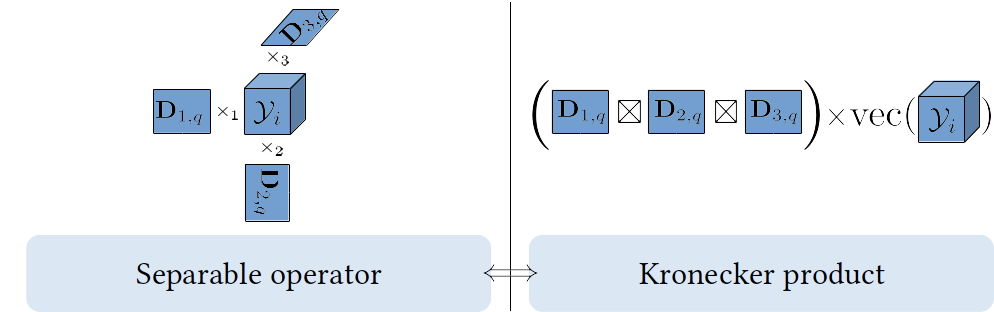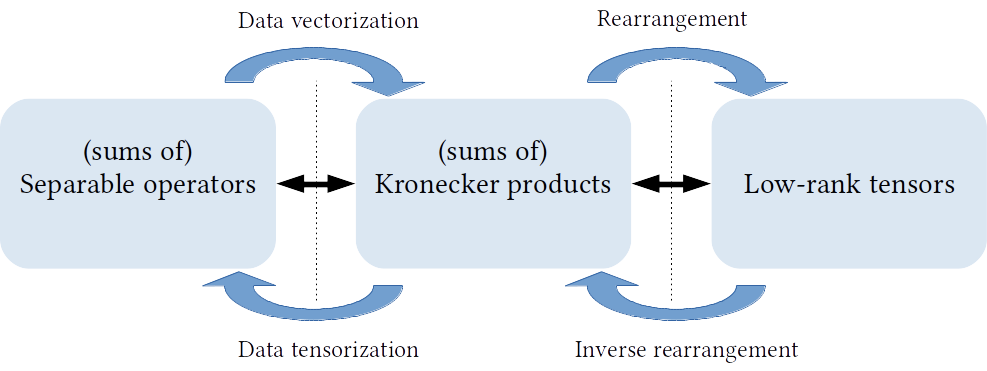# HO-SuKro

A Kronecker product of K matrices corresponds to a separable operator acting on vectorized multimodal data of order K, in the sense that each of the K operators apply independently in the corresponding mode of the K dimensional tensor data (via a tensor mode product).Additionally, such separable operators (Kronecker products) correspond to rank-1 tensors up to an isomorphism (entries rearrangement).We therefore generalize the concept of separable operators by allowing for a sum of R Kronecker products which corresponds to the more expressive class of rank-R tensor operators.We refer to the resulting sum of R Kronecker products of K factors as (R,K)-KS matrices.

Two distinct approaches for enforcing this higher-order Kronecker structure are listed below. The more recent ALS approach is the faster and more efficient one in general.

## Alternate Least Squares Approach Code

This code corresponds to the following paper:

C.F. Dantas, J.E. Cohen, and R. Gribonval. “Learning Tensor-structured Dictionaries with Application to Hyperspectral Image Denoising.” In European Signal Processing Conference (EUSIPCO), 2019.

It includes a hyperspectral image denoising demo (a more advanced hyperspectral image denoising algorithm is described here).

Related slides here and here.